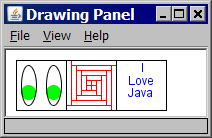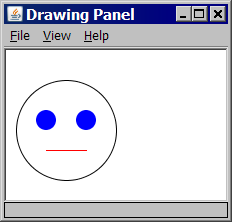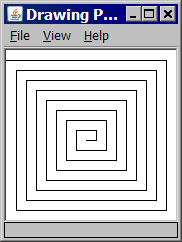# Building Java Programs, 4th edition

## Lab: Graphics

Except where otherwise noted, the contents of this document are Copyright 2013 Stuart Reges and Marty Stepp.

lab document created by Marty Stepp, Stuart Reges and Whitaker Brand

# Lab goals

Goals for this problem set:

• practice declaring and passing parameters to methods
• use the instructor-provided `DrawingPanel` and Java's `Graphics` and `Color` classes

# Graphics

Now we'll explore several exercises related to drawing graphics.• We'll use a provided class `DrawingPanel` that works with Java classes `Graphics` (a "pen" for drawing) and `Color`.
• Download `DrawingPanel.java` to your program directory.
• To check your output in the `DrawingPanel`, click File, Compare to URL....

# Parameterized methods and Graphics

When you want to divide a graphical program into multiple drawing methods, you must pass Graphics g as a parameter in addition to any other parameters. Example:

```public static void main(String[] args) {
DrawingPanel panel = new DrawingPanel(400, 300);
Graphics g = panel.getGraphics();
...
drawStuff(g, 13, 52, 7);
}

public static void drawStuff(Graphics g, int a, int b, int c) {
g.drawLine(a, 45, b, c);
...
}
```

# Exercise : Syntax errors

• The following program contains 12 mistakes! What are they? Once you think you've found them all, compile/run your corrected code.  ``` 1 2 3 4 5 6 7 8 9 10 11 12 13 14 ``` ```import Java.*; public class PrettyPicture { public static void main(String[] args) { DrawingPanel panel = DrawingPanel(220, 150); setBackgroundColor(Color.YELLOW); Graphics g = panel.Graphics(); panel.setColor(new Color.BLUE); g.drawRectangle(50, 25); g.setColor("RED"); g.fillEllipse(130, 25, 42.1, 40.5); } } ```

1. line 1: incorrect `import` statement; should import `java.awt.*`
2. line 5: missing word `new` before 2nd occurrence of `DrawingPanel`
3. line 6: method name should be `setBackground`
4. line 6: missing `panel.` before `setBackground`
5. line 8: method name should be `getGraphics`
6. line 9: the `setColor` method is part of object `g`, not `panel`
7. line 9: should not write `new` before `Color.BLUE`
8. line 10: method name should be `drawRect`
9. line 10: missing two parameters from `drawRect` (width and height)
10. line 11: color should be specified as `Color.RED`, not `"RED"`
11. line 12: method name should be `fillOval`
12. line 12: wrong parameter types; width/height must be integers

# Exercise - corrected version

• Here is a corrected version of the program:
```import java.awt.*;

public class PrettyPicture {
public static void main(String[] args) {
DrawingPanel panel = new DrawingPanel(220, 150);
panel.setBackground(Color.YELLOW);

Graphics g = panel.getGraphics();
g.setColor(Color.BLUE);
g.drawRect(50, 25, 10, 10);
g.setColor(Color.RED);
g.fillOval(130, 25, 42, 40);
}
}
```

# Exercise : FaceWrite a complete Java program that draws the following output:• window size: 220 x 150 px
• overall face circle: 100 px diameter; top-left corner at (10, 30)
• eyes: blue circles, 20 px diameter; top-left at (30, 60) and (70, 60)
• mouth: red line from (40, 100) to (80, 100)

```import java.awt.*;

public class Face1 {
public static void main(String[] args) {
DrawingPanel panel = new DrawingPanel(220, 150);
Graphics g = panel.getGraphics();

g.drawOval(10, 30, 100, 100);   // face outline
g.setColor(Color.BLUE);
g.fillOval(30, 60, 20, 20);     // eyes
g.fillOval(70, 60, 20, 20);
g.setColor(Color.RED);          // mouth
g.drawLine(40, 100, 80, 100);
}
}
```

# Exercise : Stairs loop tableConsider the output at right. The first stair's top-left corner is at position (5, 5). The first stair is 10x10 px in size. Each stair is 10px wider than the one above it.

Fill in the table below with the coordinates and sizes of the first five stairs. Note which values change and which ones stay the same.

stair x y width height
`1`
`5`
`5`
`10`
`10`
`2`
`5`
`15`
`20`
`10`
`3`
`5`
`25`
`30`
`10`
`4`
`5`
`35`
`40`
`10`
`5`
`5`
`45`
`50`
`10`

# Exercise : StairsWrite a complete Java program to draw the stairs. Copy/paste the code template below into jGRASP and fill in your own expressions or values for each stair's x, y, width, and height.

Use your table from the previous slide to help you find the correct expressions. The values that change for each stair should become expressions in terms of the loop counter variable, `i`.

```import java.awt.*;

public class Stairs1 {
public static void main(String[] args) {
DrawingPanel panel = new DrawingPanel(110, 110);
Graphics g = panel.getGraphics();
for (int i = 0; i < 10; i++) {
g.drawRect(x, y, width, height);
}
}
}
```

# Exercise : Stairs 2Modify your stairs program to draw one (or all) of the following outputs. Modify only the body in your `for` loop. (You may want to make a new table to find the expressions for x, y, width, and height.)To get each output, change the `for` loop body to the following:

```// output 2
g.drawRect(5, 5 + 10*i, 100 - 10*i, 10);
```
```// output 3
g.drawRect(95 - 10*i, 5 + 10*i, 10 + 10*i, 10);
```
```// output 4
g.drawRect(5 + 10*i, 5 + 10*i, 100 - 10*i, 10);
```

# Exercise : Face 1+2Suppose you have an existing program that draws the "face" figure at right. Let's modify the program using methods and parameters so that we can draw several faces at different locations.

continued on the next slide...

# Exercise : Face 2Modify the `Face` program to draw the following output. Write a parameterized method that draws a face at different positions.• window size: 320 x 180 px
• faces' top-left corners at (10, 30) and (150, 50)

```import java.awt.*;

public class Face2 {
public static void main(String[] args) {
DrawingPanel panel = new DrawingPanel(320, 180);
Graphics g = panel.getGraphics();
drawFace(g, 10, 30);
drawFace(g, 150, 50);
}

public static void drawFace(Graphics g, int x, int y) {
g.setColor(Color.BLACK);
g.drawOval(x, y, 100, 100);
g.setColor(Color.BLUE);
g.fillOval(x + 20, y + 30, 20, 20);
g.fillOval(x + 60, y + 30, 20, 20);
g.setColor(Color.RED);
g.drawLine(x + 30, y + 70, x + 70, y + 70);
}
}
```

# Exercise : Face 3Modify your previous Java program to draw the following output. Use a `for` loop with your parameterized method to draw faces at different positions.• window size: 520 x 180 px
• faces' top-left at (10, 30), (110, 30), (210, 30), (310, 30), and (410, 30)

```import java.awt.*;

public class Face3 {
public static void main(String[] args) {
DrawingPanel panel = new DrawingPanel(520, 180);
Graphics g = panel.getGraphics();
for (int i = 0; i < 5; i++) {
drawFace(g, 10 + i * 100, 30);
}
}

public static void drawFace(Graphics g, int x, int y) {
g.setColor(Color.BLACK);
g.drawOval(x, y, 100, 100);
g.setColor(Color.BLUE);
g.fillOval(x + 20, y + 30, 20, 20);
g.fillOval(x + 60, y + 30, 20, 20);
g.setColor(Color.RED);
g.drawLine(x + 30, y + 70, x + 70, y + 70);
}
}
```

# Exercise : SpiralWrite a Java program that draws the following output using a `for` loop.

• window size: 170 x 170 px
• "spiral" lines starting from (0, 10), going right 160, down 150, left 150, up 140, right 140, down 130, left 130, up 120, ...

```import java.awt.*;

public class Spiral {
public static void main(String[] args) {
DrawingPanel panel = new DrawingPanel(170, 170);
Graphics g = panel.getGraphics();
for (int i = 0; i < 8; i++) {
g.drawLine(      10*i,   10 + 10*i, 160 - 10*i,  10 + 10*i);  // top
g.drawLine(160 - 10*i,   10 + 10*i, 160 - 10*i, 160 - 10*i);  // right
g.drawLine( 10 + 10*i,  160 - 10*i, 160 - 10*i, 160 - 10*i);  // bottom
g.drawLine( 10 + 10*i,   20 + 10*i,  10 + 10*i, 160 - 10*i);  // left
}
}
}
```

# Exercise - answer, alternative version

```import java.awt.*;

public class Spiral {
public static void main(String[] args) {
DrawingPanel panel = new DrawingPanel(170, 170);
Graphics g = panel.getGraphics();
int x = 0, y = 10;
int len = 160;
for (int i = 0; i < 8; i++) {
g.drawLine(x, y, x + len, y);  // right
x = x + len;
len = len - 10;
g.drawLine(x, y, x, y + len);  // down
y = y + len;
g.drawLine(x, y, x - len, y);  // left
x = x - len;
len = len - 10;
g.drawLine(x, y, x, y - len);  // up
y = y - len;
}
}
}
```

# Exercise : Sierpinski Triangle

Write a Java program that draws the following output.... Just kidding!